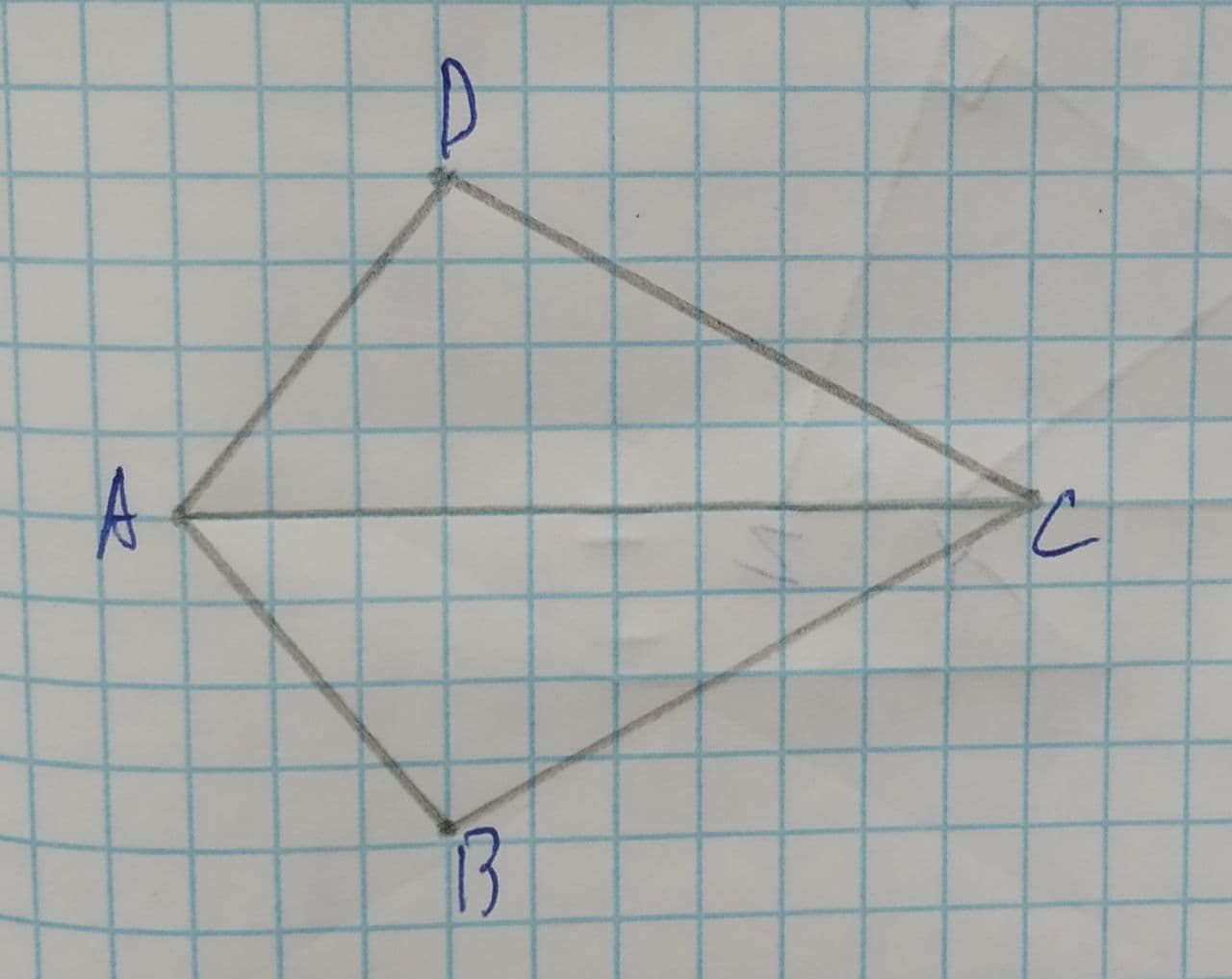Question# Prove in the following figure, triangles ABC and ADC are congruent.

Congruence
ANSWEREDTo prove: In the following figure, triangles ABC and ADC are congruent.
Given:
Figure is shown below:It is provided that $$\displaystyle\angle{B}{A}{C}=\angle{D}{A}{C}\ {\quad\text{and}\quad}\ \angle{B}{C}{A}=\angle{D}{C}{A}$$.2021-07-21
Formula used:
Angle - Side - Angle (ASA) - If two angles and the included side of one triangle are equal, respectively, to two angles and the included side of a second triangle, then the triangles are congruent.
Proof:
Make the table of statement and reason for $$\displaystyle\triangle{A}{B}{C}\stackrel{\sim}{=}\triangle{A}{D}{C}$$.
$$\displaystyle\angle{B}{A}{C}=\angle{D}{A}{C}$$ (Given)
$$\displaystyle\angle{B}{C}{A}=\angle{D}{C}{A}$$ (Given)
AC=AC (Reflexive property)
$$\displaystyle\triangle{A}{B}{C}\stackrel{\sim}{=}\triangle{A}{D}{C}$$ (ASA Congruence property)
Thus, the $$\displaystyle\triangle{A}{B}{C}\stackrel{\sim}{=}\triangle{A}{D}{C}$$ by the ASA congruence property.